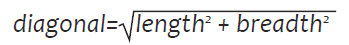# Java program to input the length and breadth of a rectangle and find its diagonal

Write a program to input the length and breadth of a rectangle and find its diagonal.`Solution`

``````import java.util.*;
class Diagonal
{
public static void main(int n)
{
Scanner sc=new Scanner(System.in);
double l,b,d;
System.out.println(“Enter the length and breadth of the rectangle:”);
l=sc.nextDouble();
b=sc.nextDouble();
d=Math.sqrt(l*l+b*b);
System.out.println(“Diagonal=”+d);
}
}``````
`Output`